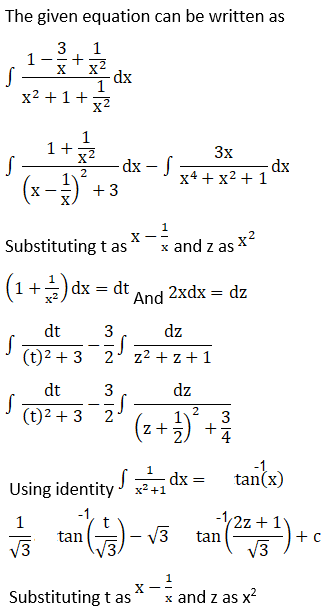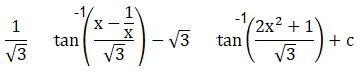# RD Sharma Solutions For Class 12 Maths Exercise 19.31 Chapter 19 Indefinite Integrals

Exercise 19.31 of Chapter 19, Indefinite Integrals consists of problems on integrals of the form $\int \frac{x^{2}+1}{x^{4}+\lambda x^{2}+1}\ dx,\int \frac{x^{2}-1}{x^{4}+\lambda x^{2}+1}\ dx$. These problems are solved by BYJU’S experts in a descriptive way to speed up the exam preparation of students. RD Sharma Solutions for Class 12 provides detailed theory with illustrations, which makes learning easy for the students. These solutions will change every student’s approach towards Mathematics and help them learn all the concepts provided in the textbook. This exercise includes eleven questions.

## Download the PDF of RD Sharma Solutions For Class 12 Chapter 19 – Indefinite Integrals Exercise 19.31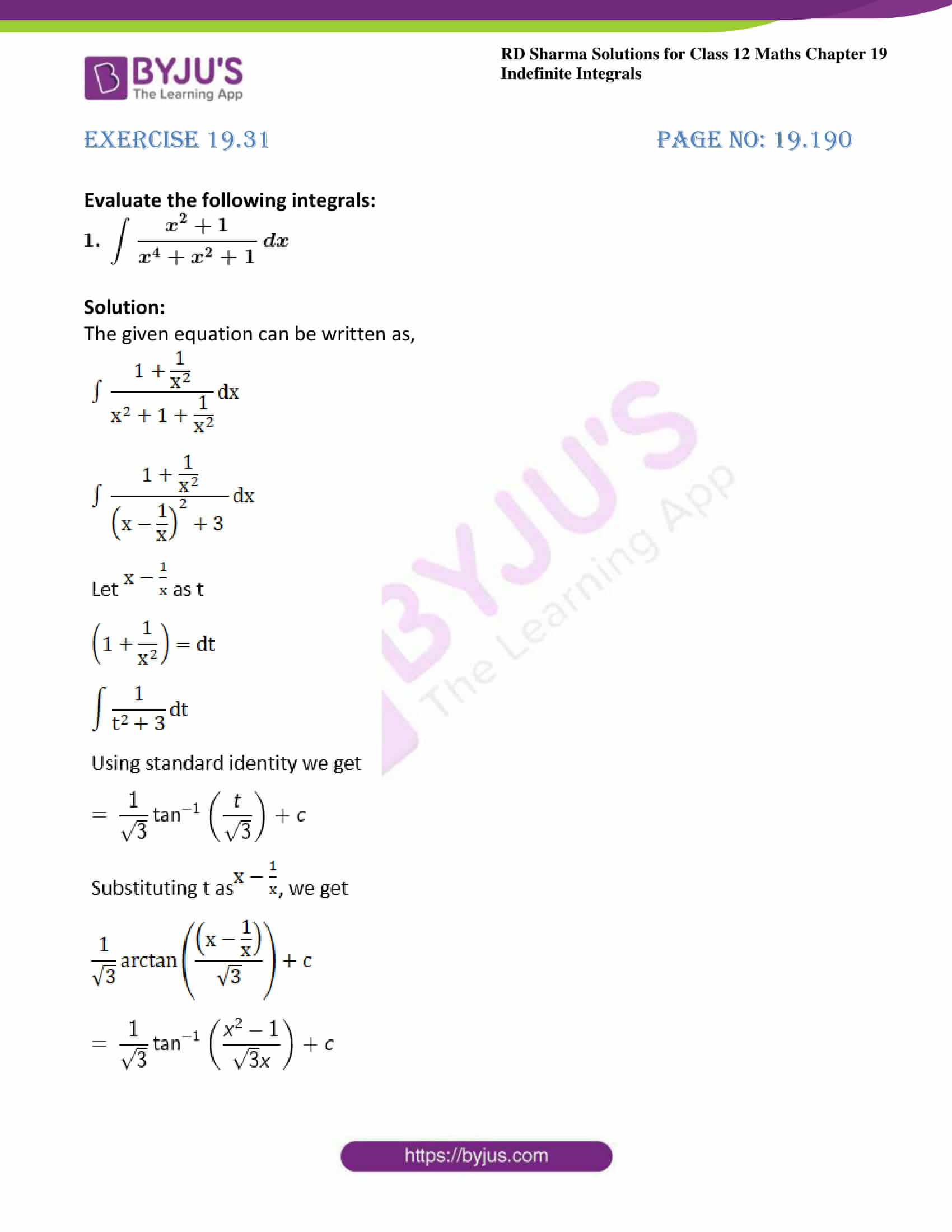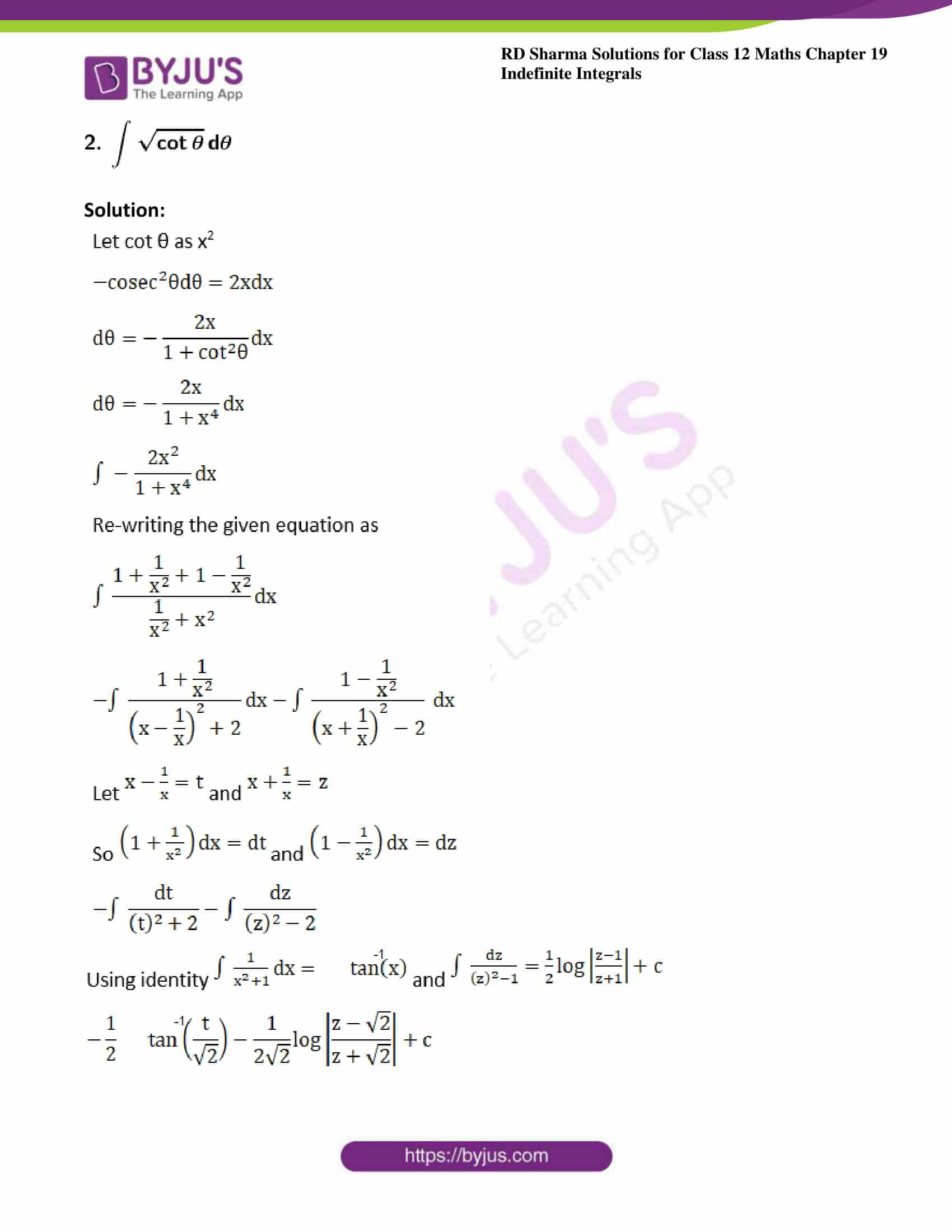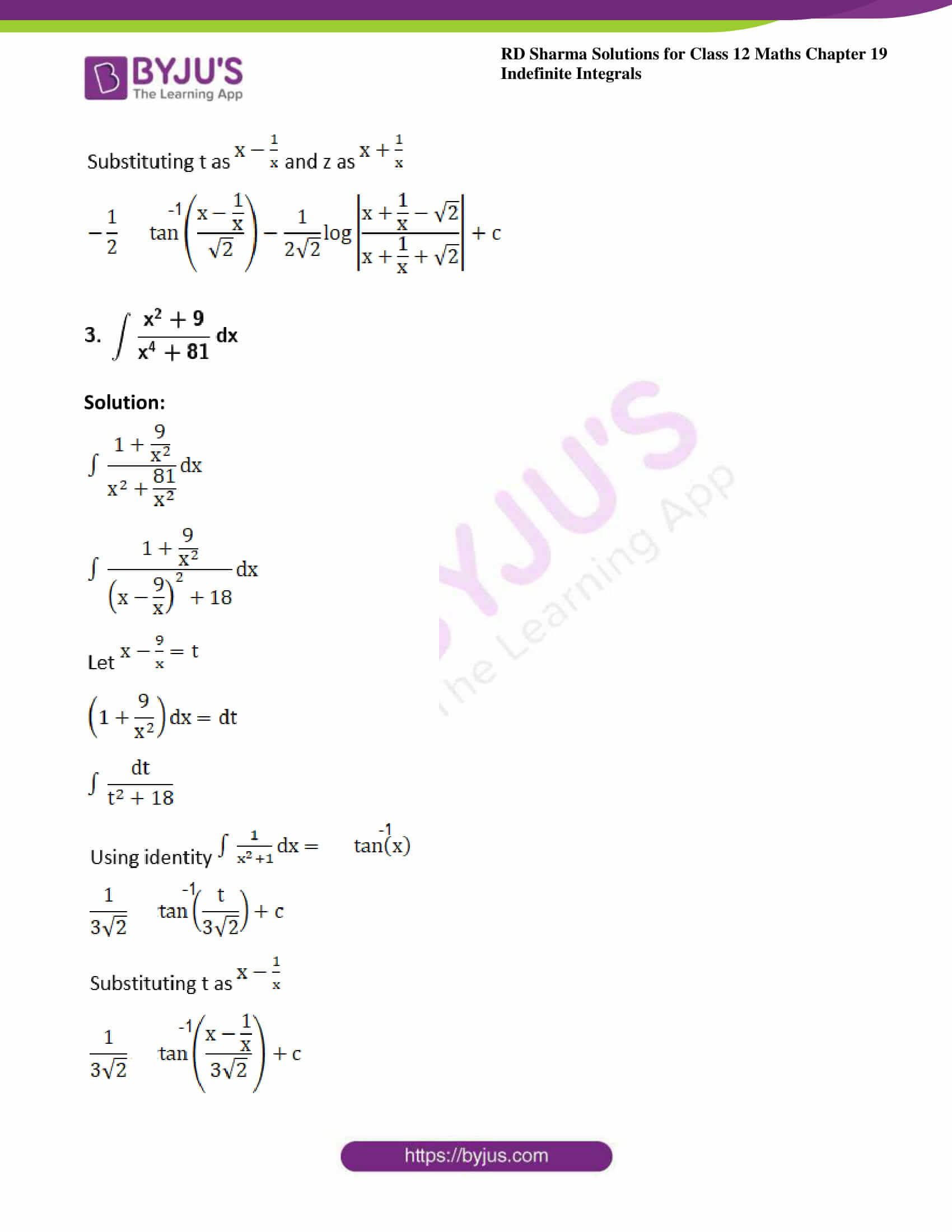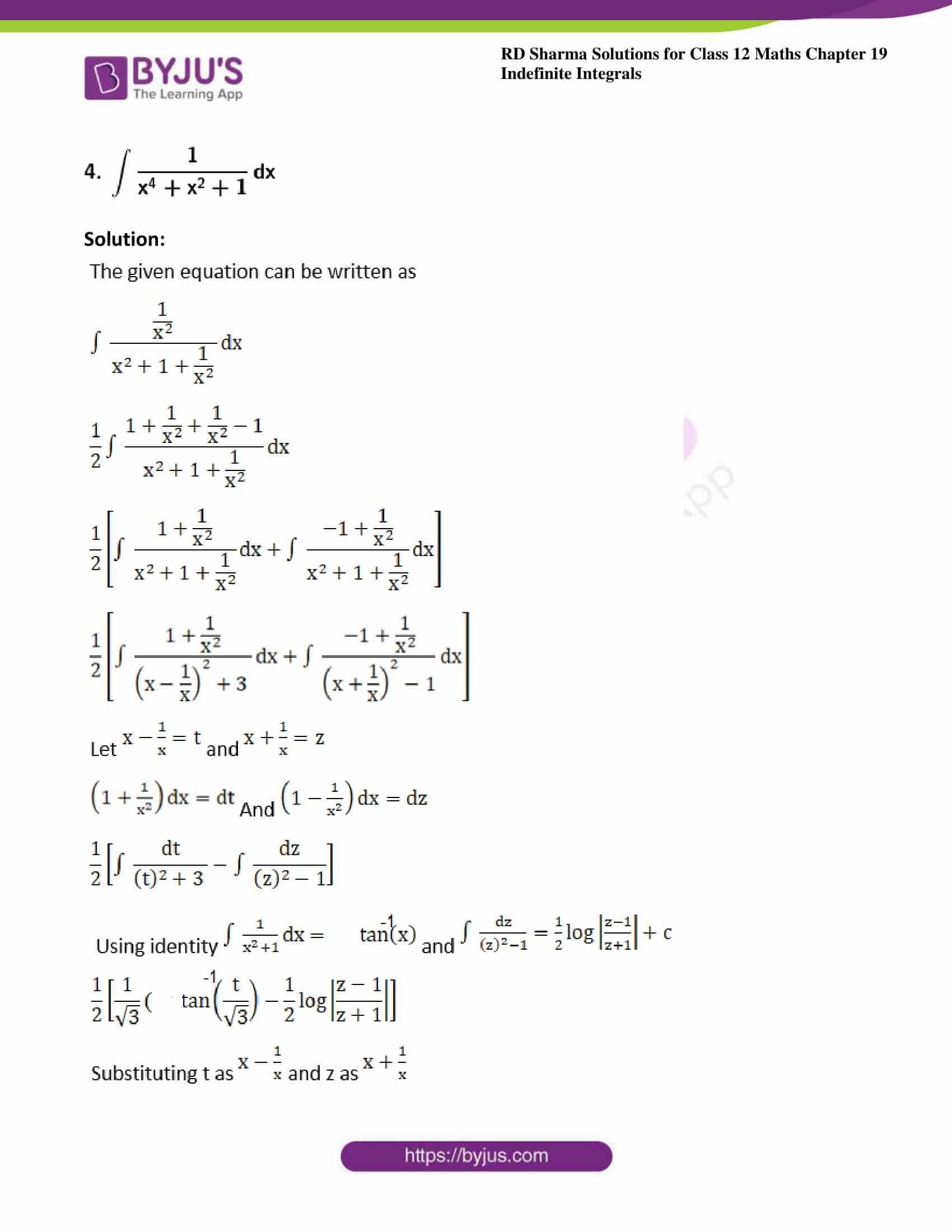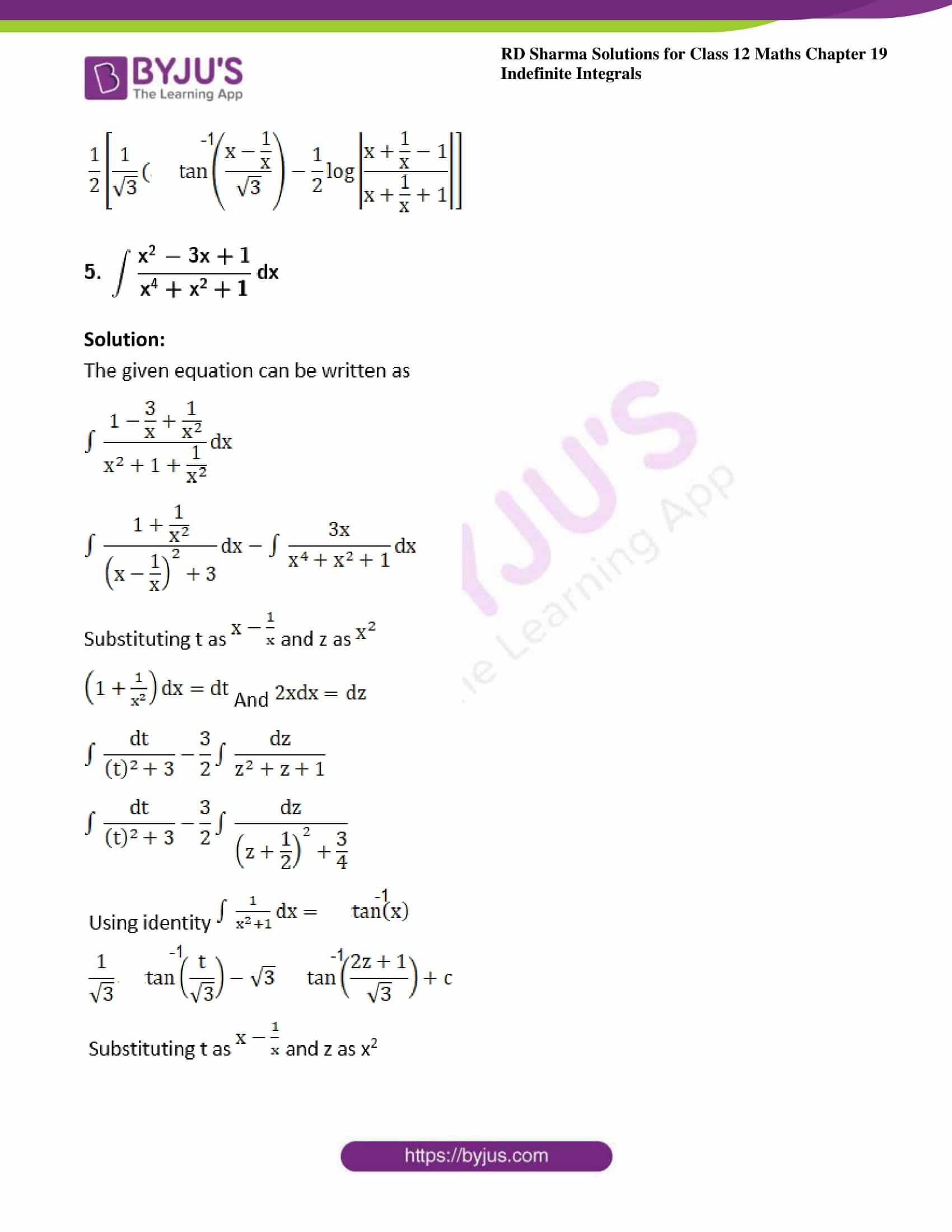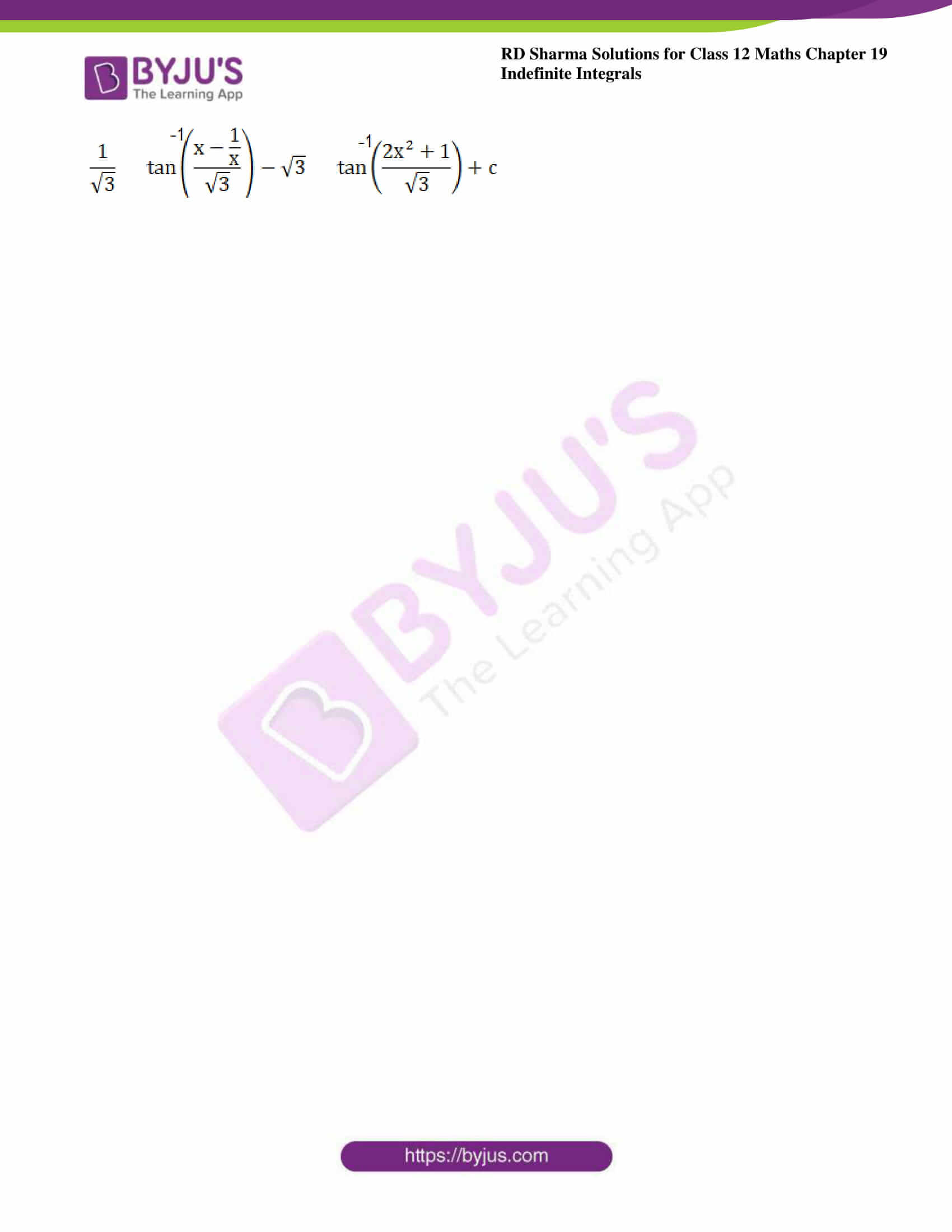### Access answers to Maths RD Sharma Solutions For Class 12 Chapter 19 – Indefinite Integrals Exercise 19.31

Evaluate the following integrals: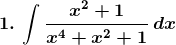Solution:

The given equation can be written as,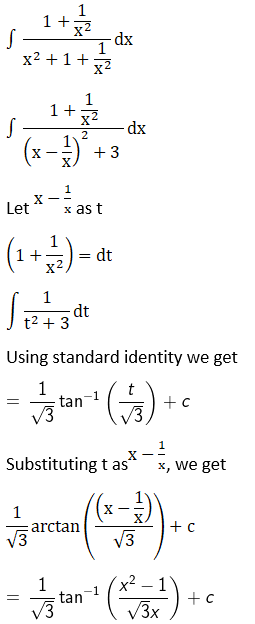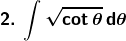Solution: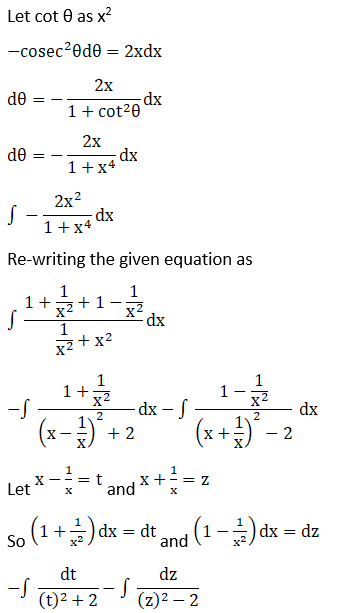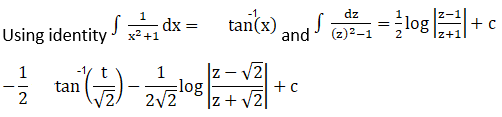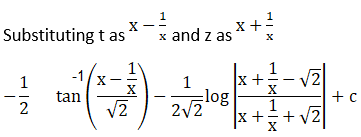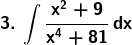Solution: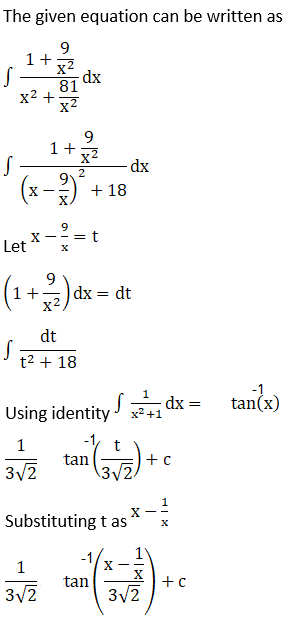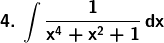Solution: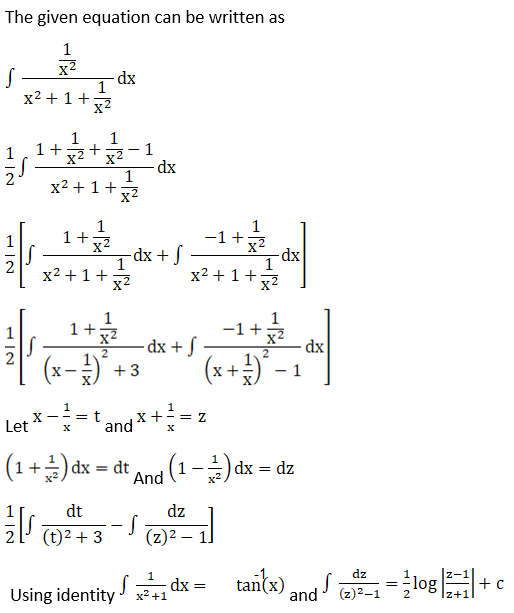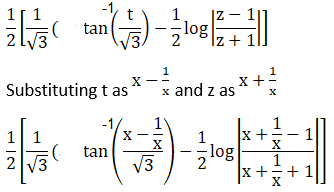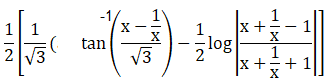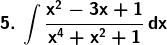Solution: